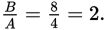# SAT Math Multiple Choice Question 261: Answer and Explanation

### Test Information

Question: 261

6.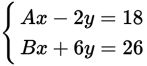If the graphs of the lines in the system of equations above intersect at (4, -1), what is the value of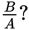• A. -3
• B.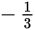• C.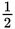• D. 2

Explanation:

D

Difficulty: Medium

Category: Heart of Algebra / Systems of Linear Equations

Strategic Advice: If the graphs intersect at (4, -1), then the solution to the system is x = 4 and y = -1. This means you can substitute these values into both equations and go from there.

Getting to the Answer: Substitute the values of x and y into each equation and solve for A and B. Then, divide B by A.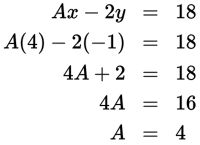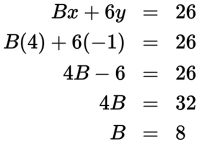Therefore,